# Pull-up Resistors

## Introduction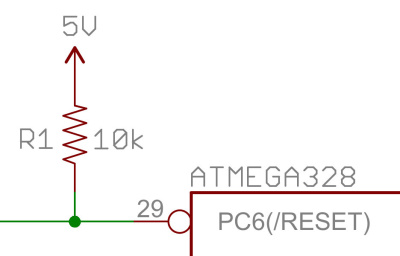Pull-up resistors are very common when using microcontrollers (MCUs) or any digital logic device. This tutorial will explain when and where to use pull-up resistors, then we will do a simple calculation to show why pull-ups are important.

## What is a Pull-up Resistor

Let’s say you have an MCU with one pin configured as an input. If there is nothing connected to the pin and your program reads the state of the pin, will it be high (pulled to VCC) or low (pulled to ground)? It is difficult to tell. This phenomena is referred to as floating. To prevent this unknown state, a pull-up or pull-down resistor will ensure that the pin is in either a high or low state, while also using a low amount of current.

For simplicity, we will focus on pull-ups since they are more common than pull-downs. They operate using the same concepts, except the pull-up resistor is connected to the high voltage (this is usually 3.3V or 5V and is often refereed to as VCC) and the pull-down resistor is connected to ground.

Pull-ups are often used with buttons and switches.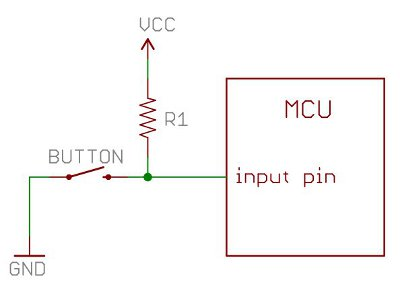With a pull-up resistor, the input pin will read a high state when the button is not pressed. In other words, a small amount of current is flowing between VCC and the input pin (not to ground), thus the input pin reads close to VCC. When the button is pressed, it connects the input pin directly to ground. The current flows through the resistor to ground, thus the input pin reads a low state. Keep in mind, if the resistor wasn’t there, your button would connect VCC to ground, which is very bad and is also known as a short.

So what value resistor should you choose?

The short and easy answer is that you want a resistor value on the order of 10kΩ for the pull-up.

A low resistor value is called a strong pull-up (more current flows), a high resistor value is called a weak pull-up (less current flows).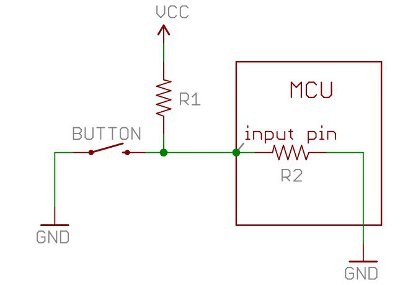The value of the pull-up resistor needs to be chosen to satisfy two conditions:

1. When the button is pressed, the input pin is pulled low. The value of resistor R1 controls how much current you want to flow from VCC, through the button, and then to ground.
2. When the button is not pressed, the input pin is pulled high. The value of the pull-up resistor controls the voltage on the input pin.

For condition 1, you don’t want the resistor’s value too low. The lower the resistance, the more power will be used when the button is hit. You generally want a large resistor value (10kΩ), but you don’t want it too large as to conflict with condition 2. A 4MΩ resistor might work as a pull-up, but its resistance is so large (or weak) that it may not do its job 100% of the time.

The general rule for condition 2 is to use a pull-up resistor (R1) that is an order of magnitude (1/10th) less than the input impedance (R2) of the input pin. An input pin on a microcontroller has an impedance that can vary from 100k-1MΩ. For this discussion, impedance is just a fancy way of saying resistance and is represented by R2 in the picture above. So, when the button is not pressed, a very small amount of current flows from VCC through R1 and into the input pin. The pull-up resistor R1 and input pin impedance R2 divides the voltage, and this voltage needs to be high enough for the input pin to read a high state.

For example, if you use a 1MΩ resistor for the pull-up R1 and the input pin’s impedance R2 is on the order of 1MΩ (forming a voltage divider), the voltage on the input pin is going to be around half of VCC, and the microcontroller might not register the pin being in a high state. On a 5V system, what does the MCU read on the input pin if the voltage is 2.5V? Is it a high or a low? The MCU doesn’t know and you might read either a high or a low. A resistance of 10k to 100kΩ for R1 should avoid most problems.

Since pull-up resistors are so commonly needed, many MCUs, like the ATmega328 microcontroller on the Arduino platform, have internal pull-ups that can be enabled and disabled. To enable internal pull-ups on an Arduino, you can use the following line of code in your setup() function:

``````pinMode(5, INPUT_PULLUP); // Enable internal pull-up resistor on pin 5
``````

Another thing to point out is that the larger the resistance for the pull-up, the slower the pin is to respond to voltage changes. This is because the system that feeds the input pin is essentially a capacitor coupled with the pull-up resistor, thus forming a RC filter, and RC filters take some time to charge and discharge. If you have a really fast changing signal (like USB), a high value pull-up resistor can limit the speed at which the pin can reliably change state. This is why you will often see 1k to 4.7KΩ resistors on USB signal lines.

All of these factors play into the decision on what value pull-up resistor to use.

## Calculating a Pull-up Resistor ValueLet’s say you want to limit the current to approximately 1mA when the button is pressed in the circuit above, where Vcc = 5V. What resistor value should you use?

It is easy to show how to calculate the pull-up resistor using Ohm’s Law: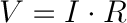Referring to the schematic above, Ohm’s Law now is: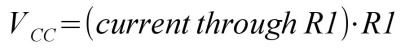Rearrange the above equation with some simple algebra to solve for the resistor:Remember to convert all of your units into volts, amps and Ohms before calculating (e.g. 1mA = 0.001 Amps). The solution is to use a 5kΩ resistor.

Now you should be familiar with what a pull-up resistor is and how it works.